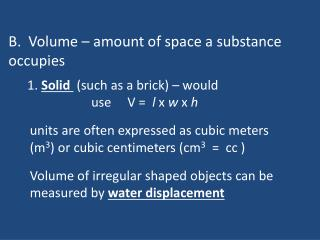Download PresentationB. Volume – amount of space a substance occupies

# B. Volume – amount of space a substance occupies - PowerPoint PPT PresentationDownload Presentation## B. Volume – amount of space a substance occupies

- - - - - - - - - - - - - - - - - - - - - - - - - - - E N D - - - - - - - - - - - - - - - - - - - - - - - - - - -
##### Presentation Transcript

1. B. Volume – amount of space a substance occupies 1. Solid (such as a brick) – would use V = l x w x h units are often expressed as cubic meters (m3) or cubic centimeters (cm3 = cc ) Volume of irregular shaped objects can be measured by water displacement

2. 2. Liquid - measure capacity in a container. Most common units are ml and liters. An important conversion is: 1 mL = 1 cm3 = 1 cc

3. Ways to measure liquids: Graduated Cylinder accurate device

4. b) Pipettes – accurate device

5. Beaker and flask not as accurate

6. C. Mass (kg)– measure of the amount of matter in an object Ex: golf ball vs. ping pong ball Each have about the same volume but mass is different. Golf ball has about 18x more mass

7. Measuring mass: 1. Triple balance beam 2. Digital scales

8. Mass vs. Weight Mass is amount of matter in an object. Weight is the measure of gravity’s effect on something (force) units are Newtons (N) for metric and pound (lbs) for English system. Ex: move to a different planet and weight changes but mass stays the same weightlessness not masslessness (not even a word !!)

9. D. Temperature – measure of the amount of molecular movement Important Temperatures to Memorize

10. Measuring temperature: 1. Mercury thermometers 2. Digital Thermometers

11. E. Time (seconds)– duration; Measuring devices: 1. stopwatch 2. digital timers

12. F. Mole – the amount of a substance (mol) mole = 6.022 x 1023 particles of a substance Ex: a mole of popcorn = 6.022 x 1023 of kernels of popcorn Enough popcorn to cover the entire US at a height of 310 ft!

13. The mole is showing the value of a mole Happy mole day….October 23rd!

14. www.moleday.org